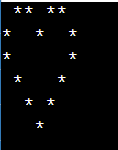Dark Mode On/Off

# C++ Program To Print Heart Pattern Program Using Star

In this tutorial, we will see how to print star patterns using * in the c++ program.C++ Heart Pattern made by Control statement, Program will print the Heart pattern according to the user input meaning that heart size will depend on input values, for good heart patter use numbers 4- 8 according to Turbo monitor. If the user input is 0 then the program will exit while the input value is an odd type then first we make this value as an event type for the perfect design.

## C++ Program To Print Heart Pattern

``````#include<iostream>
using namespace std;

int main()
{

int n,row,col;
n = 6;
for(row=0;row<n;row++)
{
for(col=0;col<n+1;col++)
if ((row==0 && col%3!=0) || (row==1 && col%3==0) || (row-col==2) || (row+col==8))
cout<<("*");
else
cout<<(" ");

cout<<("\n");

}
return 0;
}``````## Conclusion

Here we have seen how to implement the print the c++ program heart-shaped pattern using *

Want to learn coding?
Try our new interactive courses.
Over 20,000+ students enrolled.